Courses

# Test: Transfer Function

## 20 Questions MCQ Test RRB JE for Electronics & Communication Engineering | Test: Transfer Function

Description
This mock test of Test: Transfer Function for Electronics and Communication Engineering (ECE) helps you for every Electronics and Communication Engineering (ECE) entrance exam. This contains 20 Multiple Choice Questions for Electronics and Communication Engineering (ECE) Test: Transfer Function (mcq) to study with solutions a complete question bank. The solved questions answers in this Test: Transfer Function quiz give you a good mix of easy questions and tough questions. Electronics and Communication Engineering (ECE) students definitely take this Test: Transfer Function exercise for a better result in the exam. You can find other Test: Transfer Function extra questions, long questions & short questions for Electronics and Communication Engineering (ECE) on EduRev as well by searching above.
QUESTION: 1

Solution:
QUESTION: 2

### Which of the following is not the feature of modern control systems?

Solution:

For a good control system the speed of response and stability must be high and for the slow and sluggish response is not used and undesirable.

QUESTION: 3

### The principles of homogeneity and superposition are applied to ______.

Solution:

Superposition theorem states that for two signals additivity and homogeneity property must be satisfied and that is applicable for the LTI systems.

QUESTION: 4

The sum of the gains of the feedback paths in the signal flow graph shown in fig.  is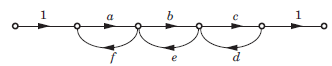Solution:
QUESTION: 5

A control system whose step response is -0.5(1+e-2t) is cascaded to another control block whose impulse response is e-t. What is the transfer function of the cascaded combination?

Solution:
• Let h1(t) is the impulse response of system.
h1(t) = d/dt(-0.5(1+e-2t)) h1(t) = e(-2t) h2(t)  = e(-t)
• Cascading of two system equals to the convolution of both and convolution in time domain is equals to multiplication in frequency domain.
• So by taking Laplace transform of above two
H1(s) =1/(s+2), H2(s) = 1/(s+1)
• Hence the transfer function of cascaded system is
H(s) = H1(s)H2(s)
H(s)= 1/(s+1)(s+2)
QUESTION: 6

The overall transfer function C/R of the system shown in fig. will be: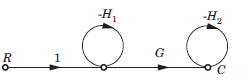Solution: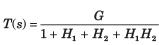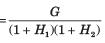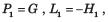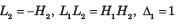QUESTION: 7

Consider the signal flow graphs shown in fig. The transfer 2 is of the graph: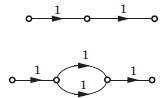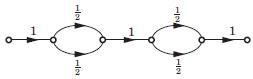Solution:

There are no loop in any graph. So option (B) is correct.QUESTION: 8

Consider the List I and List II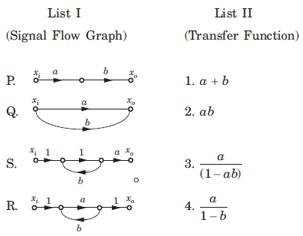The correct match is

Solution:

P. P1 = ab, Δ = 1, L = 0 ,T = ab
Q1 P1 = a, P2 = 6 , Δ = 1, L = Δk = 0,T  = a+b
R. P1 = a, L1 = b, Δ = 1 - b, Δ1 =1,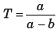S. P1 = a, L1 = ab, Δ = 1 - ab, Δ1 = 1,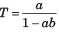QUESTION: 9

For the signal flow graph shown in fig. an equivalent graph is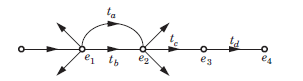Solution:
QUESTION: 10

Consider the block diagram shown in figure.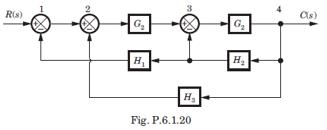For this system the signal flow graph is

Solution:

Option (A) is correct. Best method is to check the signal flow graph. In block diagram there is feedback from 4 to 1 of gain - H1H2 . The signal flow graph of option (A) has feedback from 4 to 1 of gain - H1H2

QUESTION: 11

The block diagram of a system is shown in fig. The closed loop transfer function of this system is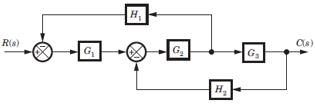Solution:

Consider the block diagram as SFG. There are two feedback loop -G1G2H1 and -G2G3H2 and one forward path G1G2 G3 . So (D) is correct option.

QUESTION: 12

For the system shown in fig. transfer function C(s) R(s) is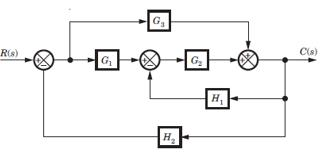Solution:

Consider the block diagram as a SFG. Two forward path G1G2 and G3 and three loops -G1G2 H2, -G2H1, -G3 H2
There are no nontouching loop. So (B) is correct.

QUESTION: 13

In the signal flow graph shown in fig. the transfer function is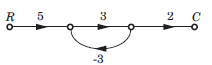Solution:

P1 = 5 x 3 x 2 = 30, Δ = 1 - (3x - 3) = 10
Δ1 = 1,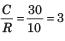QUESTION: 14

In the signal flow graph shown in fig. the gain C/R is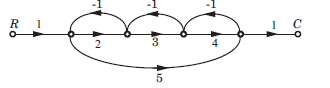Solution:

P1 = 2 x 3 x 4 = 24 , P2 = 1 x 5 x 1 = 5
L1 = -2, L2 = -3, L3 = -4, L4 = -5,
L1L3 = 8, Δ = 1 -(-2 - 3 - 4 - 5) + 8 = 23, Δ1 = 1, Δ2 = 1 - (-3) = 4,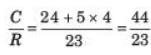QUESTION: 15

The gain C(s)/R(s) of the signal flow graph shown in fig.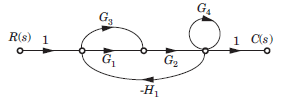Solution:
QUESTION: 16

The negative feedback closed-loop system was subjected to 15V. The system has a forward gain of 2 and a feedback gain of 0.5. Determine the output voltage and the error voltage.

Solution:

Given:
G(s) = 2
H(s) = 0.5 and R(s) = 10V
Output voltage: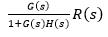= (2/1 + 2 x 0.5) x 15 = 15V
Error voltage: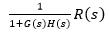= (1/1 + 2 x 0.5) x 15 = 7.5V

QUESTION: 17

For the block diagram shown in fig. transfer function C(s)/R(s) is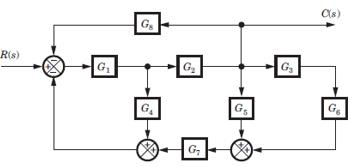Solution:

Four loops -G1G4, -G1G2G5, -G1,G2G5G7 and -G1G2G3G3G7.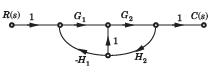There is no nontouching loop. So (B) is correct.

QUESTION: 18

For the block diagram shown in fig. the numerator of transfer function is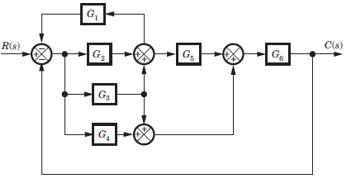Solution:

SFG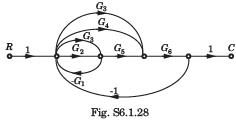P1 = G2G5G6 , P2 = G3G5G6, P3 = G3G6 , P4 = G4G6
If any path is deleted, there would not be any loop.
Hence Δ1 = Δ2 = Δ3 = Δ4 = 1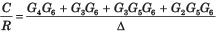QUESTION: 19

For the block diagram shown in fig.  the transfer function C(s)/R(s) is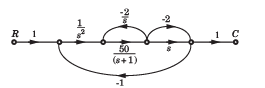Solution: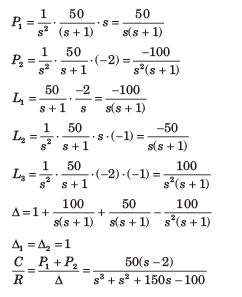QUESTION: 20

In the signal flow graph of figure y/x equals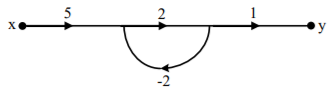Solution:

Transfer function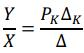PK = 5 x 2 x 1 = 10
ΔK = 1
Δ = 1 - (-4) = 5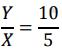= 2